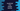# How to find simple interest with JavaScript## How to find Simple Interest with JavaScript:

Let’s learn how to calculate and print the simple interest in JavaScript with examples. The program will take the values as inputs from the user and print the calculated Simple Interest.

### How to calculate Simple Interest:

To calculate the simple interest, we need to get the pricipal amount, rate of interest, and the time period in years to calculate the interest. If `P`, `r`, and `n` are the values of the principal amount, rate of interest, and time-period, we can calculate the simple interest by using the below formula:

``Simple Interest = (P * n * r)/100``

We need these three values as inputs from the user and we can calculate the simple interest.

### Method 1: By using predefined values:

In this method, we will use predefined principal amount, interest rate, and time period values. The program will calculate the result with the above formula.

``````const p = 1200;
const n = 5;
const r = 0.2;

const simpleInterest = (p * n * r) / 100;

console.log(`Simple Interest: \${simpleInterest}`);``````

Here, we are using predefined values for `p`, `n`, and `r`. The calculated simple interest is assigned to the `simpleInterest` variable. If you run this program, it will print the calculated simple interest value.

``Simple Interest: 12``

### Method 2: By using user-input values:

The following example will take the values as inputs from the user and print the calculated simple interest. Create one file `index.html` and add the below code to it:

``````<!DOCTYPE html>
<html lang="en">
<script>
function calculateSI() {
let p = document.getElementById("inputP").value;
let n = document.getElementById("inputN").value;
let r = document.getElementById("inputR").value;

let simpleInterest = (p * n * r) / 100;

document.getElementById("result").innerHTML = simpleInterest;
}
</script>
<body>
<h4>Enter the principal amount:</h4>
<input id="inputP" />
<h4>Enter the rate of interest:</h4>
<input id="inputR" />
<h4>Enter the time:</h4>
<input id="inputN" />
<br /><br />
<button onclick="calculateSI()">Calculate Simple Interest</button>
<p id="result"></p>
</body>
</html>``````

Here,

• We have three input fields to take the principal value, rate of interest, and time.
• There is one button, on clicking this button, it calls the `calculateSI` function. This function calculates the simple interest with the user-provided value.
• Inside the function, it reads the value of the principal amount, interest rate, and the number of years and assigns these values to three different variables.
• It calculates the simple interest value and assigns it to the `simpleInterest` variable.
• This value is assigned to the `p` component `result`.

If you open this file in a browser, it will look as below: